HomePuzzle ContestSingle Shift PuzzleFlip Flat RubikDouble Shift PuzzleRotate 3x3 PuzzleRotate 5x5 PuzzleRotate 7x7 PuzzleNine Faces RubikSix Faces Rubik24 Faces Magic Cube4D Rubik CubeQuotes By AuthorQuotes By SubjectQuote Of The DayDetective MysteryReversiLogic TestMath TestWord Of The DayUnsolved ProblemsHall of FameContact UsLoginSign UpSign OutMy AccountChange PasswordAbout UsContributorsLink To UsPrivacy PolicyTerms of ServiceSite Map

## 24 Faces Flat Magic Cube PuzzleThe 24 Faces Flat Magic Cube Puzzle is a two dimensional variation of Magic Cube 4D puzzle. Magic Cube 4D is a four-dimensional analog of Rubik's cube.

The Puzzle consists of 8x6 moving colored bocks. All moving blocks are marked with two digits. First digit represents a 3x3 square id. Second digit represents a block id inside the 3x3 square. Each block has a mirror block. 48 blocks make 24 pairs (faces). For example, the block 2.1 is a mirror of the block 1.2. If the block 2.1 replaces the block 2.3, the mirror block makes a similar move and the block 1.2 replace the block 3.2.
Click on any block marked by two digits to shift blokes. Each move shifts 4 blocks inside the selected 3x3 square and 4 mirror blocks. Similar to Six Faces and Nine Faces puzzles, the 24 Faces puzzle shifts elements inside selected 3x3 square that forms one of the bloke's angles. Any shift is always clockwise. Left and right edges of each 3x3 square are linked. When right block shifts to the right it appears on the same row in the left corner. Similar rule applies to the top and the bottom edges.
For example, if you click on a “1.2” block, it will shift Clockwise 4 elements (see Figure 1).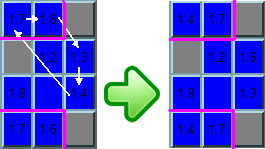Figure 1
The same move will shift four mirror blokes in the same order (7.1 => 6.1 => 3.1 => 4.1 => 7.1). Figure 2 shows the complete move.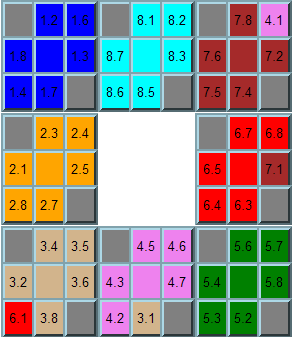Figure 2
In the example above we have rotated blue blocks and the mirror blocks shifted in the following order: the Red block has moved to the Tan area, Tan to Violet, Violet to Brown, and Brown to Red. The 24 Faces Flat puzzle is a two dimensional analog of the Magic Cube 4D puzzle. This move is similar to the Magic Cube 4D puzzle move shown in Figure 3. Puzzles are not completely equal. Magic Cube 4D puzzle rotates a layer while 24 Faces puzzle rotates the surface only.Figure 3
For the next example, let's click on the “1.3” block. The move is presented on Figures 4, 5, and 6.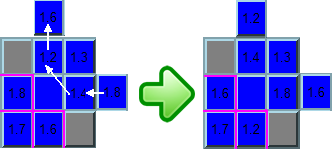Figure 4
The same move will shift four mirror blokes in the same order (6.1 => 8.1 => 4.1 => 2.1 => 6.1). Figure 5 shows the complete move.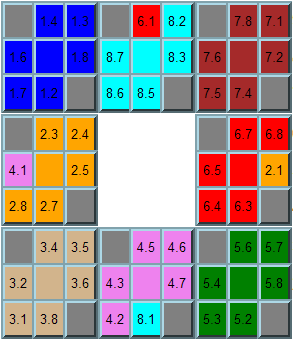Figure 5
In the example above we have rotated blue blocks and the mirror blocks shifted in the following order: the Red block has moved to the Cyan area, Cyan to Violet, Violet to Orange, and Orange to Red. This move is similar to the Magic Cube 4D puzzle move shown in Figure 6.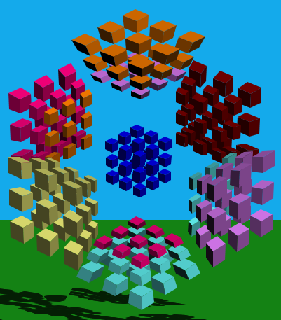Figure 6
For the next example let's click on “1.4” block. The move is presented on Figures 7, 8, and 9.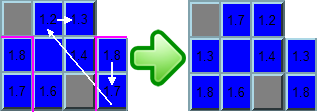Figure 7
The same move will shift four mirror blokes in the same order (6.1 => 8.1 => 4.1 => 2.1 => 6.1). The complete move is shown on Figure 8.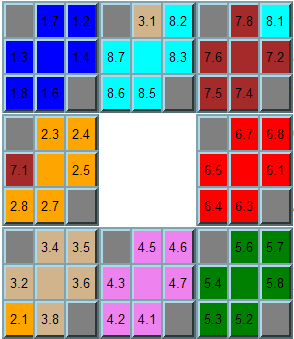Figure 8
In the example above we have rotated blue blocks and the mirror blocks shifted in the following order: Red block has moved to the Cyan area, Cyan to Violet, Violet to Orange, and Orange to Red. This move is similar to the Magic Cube 4D puzzle move shown in Figure 9.Figure 9
The click on the block 1.8 moves the same blocks as 1.2 in the opposite direction. Similar, the click on the block 1.7 moves the same blocks as 1.3 in the opposite direction, and the click on the block 1.6 moves the same blocks as 1.4 in the opposite direction.